Publicité

# Types of Language in Theory of Computation

30 Mar 2017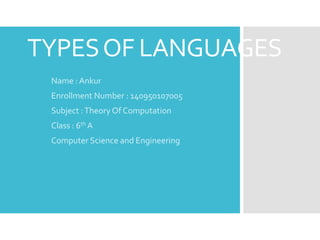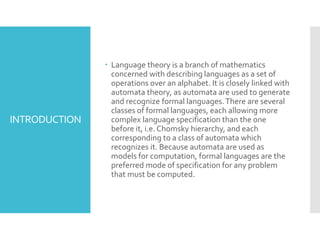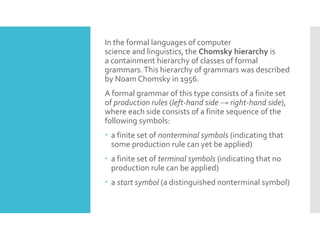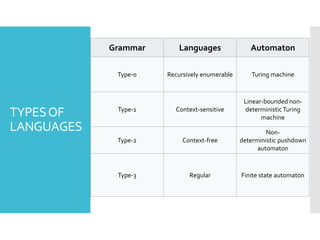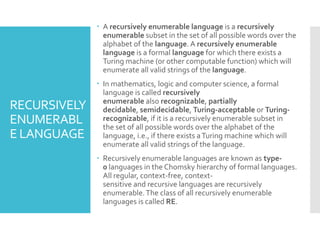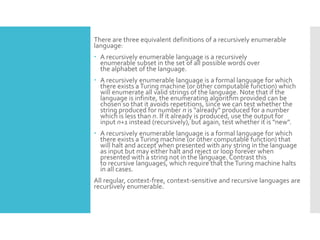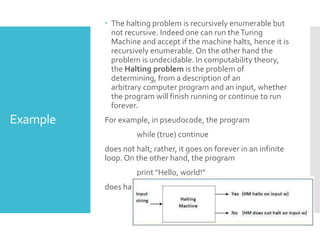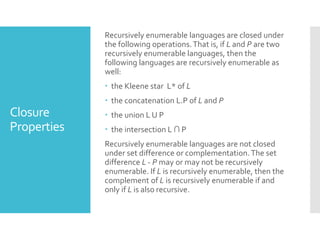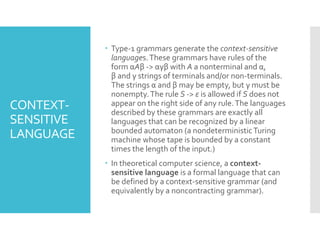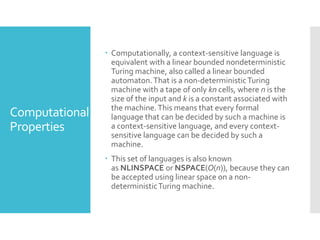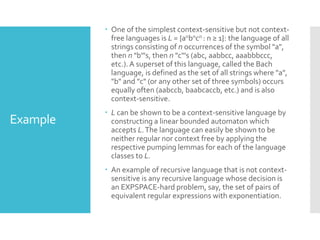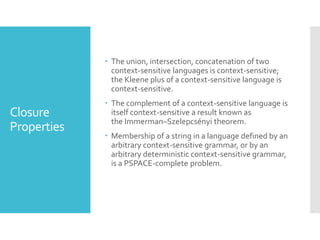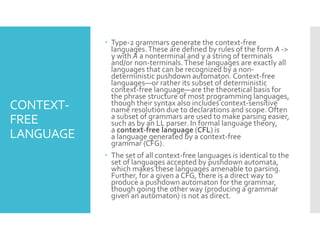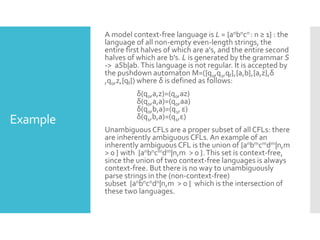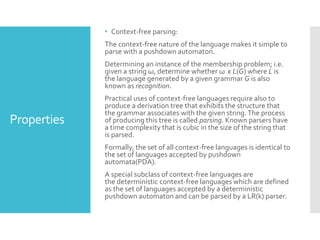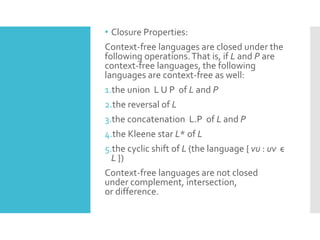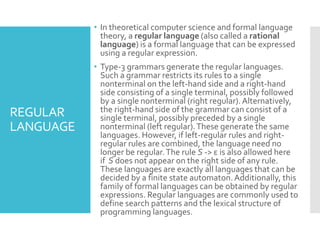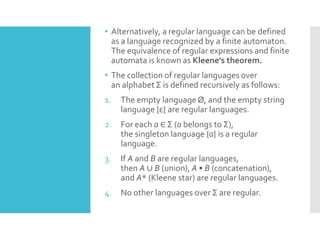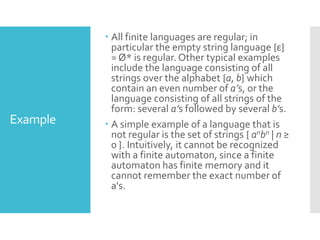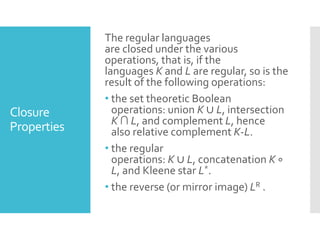1 sur 21
Publicité

### Types of Language in Theory of Computation

1. TYPESOF LANGUAGES Name : Ankur Enrollment Number : 140950107005 Subject :Theory Of Computation Class : 6th A Computer Science and Engineering
2. INTRODUCTION  Language theory is a branch of mathematics concerned with describing languages as a set of operations over an alphabet. It is closely linked with automata theory, as automata are used to generate and recognize formal languages.There are several classes of formal languages, each allowing more complex language specification than the one before it, i.e. Chomsky hierarchy, and each corresponding to a class of automata which recognizes it. Because automata are used as models for computation, formal languages are the preferred mode of specification for any problem that must be computed.
3. In the formal languages of computer science and linguistics, the Chomsky hierarchy is a containment hierarchy of classes of formal grammars.This hierarchy of grammars was described by Noam Chomsky in 1956. A formal grammar of this type consists of a finite set of production rules (left-hand side → right-hand side), where each side consists of a finite sequence of the following symbols:  a finite set of nonterminal symbols (indicating that some production rule can yet be applied)  a finite set of terminal symbols (indicating that no production rule can be applied)  a start symbol (a distinguished nonterminal symbol)
4. TYPESOF LANGUAGES Grammar Languages Automaton Type-0 Recursively enumerable Turing machine Type-1 Context-sensitive Linear-bounded non- deterministicTuring machine Type-2 Context-free Non- deterministic pushdown automaton Type-3 Regular Finite state automaton
5. RECURSIVELY ENUMERABL E LANGUAGE  A recursively enumerable language is a recursively enumerable subset in the set of all possible words over the alphabet of the language. A recursively enumerable language is a formal language for which there exists a Turing machine (or other computable function) which will enumerate all valid strings of the language.  In mathematics, logic and computer science, a formal language is called recursively enumerable also recognizable, partially decidable, semidecidable, Turing-acceptable or Turing- recognizable, if it is a recursively enumerable subset in the set of all possible words over the alphabet of the language, i.e., if there exists aTuring machine which will enumerate all valid strings of the language.  Recursively enumerable languages are known as type- 0 languages in the Chomsky hierarchy of formal languages. All regular, context-free, context- sensitive and recursive languages are recursively enumerable.The class of all recursively enumerable languages is called RE.
6. There are three equivalent definitions of a recursively enumerable language:  A recursively enumerable language is a recursively enumerable subset in the set of all possible words over the alphabet of the language.  A recursively enumerable language is a formal language for which there exists aTuring machine (or other computable function) which will enumerate all valid strings of the language. Note that if the language is infinite, the enumerating algorithm provided can be chosen so that it avoids repetitions, since we can test whether the string produced for number n is "already" produced for a number which is less than n. If it already is produced, use the output for input n+1 instead (recursively), but again, test whether it is "new".  A recursively enumerable language is a formal language for which there exists aTuring machine (or other computable function) that will halt and accept when presented with any string in the language as input but may either halt and reject or loop forever when presented with a string not in the language. Contrast this to recursive languages, which require that theTuring machine halts in all cases. All regular, context-free, context-sensitive and recursive languages are recursively enumerable.
7. Example  The halting problem is recursively enumerable but not recursive. Indeed one can run theTuring Machine and accept if the machine halts, hence it is recursively enumerable. On the other hand the problem is undecidable. In computability theory, the Halting problem is the problem of determining, from a description of an arbitrary computer program and an input, whether the program will finish running or continue to run forever. For example, in pseudocode, the program while (true) continue does not halt; rather, it goes on forever in an infinite loop. On the other hand, the program print "Hello, world!“ does halt.
8. Closure Properties Recursively enumerable languages are closed under the following operations.That is, if L and P are two recursively enumerable languages, then the following languages are recursively enumerable as well:  the Kleene star L* of L  the concatenation L.P of L and P  the union L U P  the intersection L ∩ P Recursively enumerable languages are not closed under set difference or complementation.The set difference L - P may or may not be recursively enumerable. If L is recursively enumerable, then the complement of L is recursively enumerable if and only if L is also recursive.
9. CONTEXT- SENSITIVE LANGUAGE  Type-1 grammars generate the context-sensitive languages.These grammars have rules of the form αAβ -> αγβ with A a nonterminal and α, β and γ strings of terminals and/or non-terminals. The strings α and β may be empty, but γ must be nonempty.The rule S -> ε is allowed if S does not appear on the right side of any rule.The languages described by these grammars are exactly all languages that can be recognized by a linear bounded automaton (a nondeterministicTuring machine whose tape is bounded by a constant times the length of the input.)  In theoretical computer science, a context- sensitive language is a formal language that can be defined by a context-sensitive grammar (and equivalently by a noncontracting grammar).
10. Computational Properties  Computationally, a context-sensitive language is equivalent with a linear bounded nondeterministic Turing machine, also called a linear bounded automaton.That is a non-deterministicTuring machine with a tape of only kn cells, where n is the size of the input and k is a constant associated with the machine.This means that every formal language that can be decided by such a machine is a context-sensitive language, and every context- sensitive language can be decided by such a machine.  This set of languages is also known as NLINSPACE or NSPACE(O(n)), because they can be accepted using linear space on a non- deterministicTuring machine.
11. Example  One of the simplest context-sensitive but not context- free languages is L = {anbncn : n ≥ 1}: the language of all strings consisting of n occurrences of the symbol "a", then n "b"'s, then n "c"'s (abc, aabbcc, aaabbbccc, etc.). A superset of this language, called the Bach language, is defined as the set of all strings where "a", "b" and "c" (or any other set of three symbols) occurs equally often (aabccb, baabcaccb, etc.) and is also context-sensitive.  L can be shown to be a context-sensitive language by constructing a linear bounded automaton which accepts L.The language can easily be shown to be neither regular nor context free by applying the respective pumping lemmas for each of the language classes to L.  An example of recursive language that is not context- sensitive is any recursive language whose decision is an EXPSPACE-hard problem, say, the set of pairs of equivalent regular expressions with exponentiation.
12. Closure Properties  The union, intersection, concatenation of two context-sensitive languages is context-sensitive; the Kleene plus of a context-sensitive language is context-sensitive.  The complement of a context-sensitive language is itself context-sensitive a result known as the Immerman–Szelepcsényi theorem.  Membership of a string in a language defined by an arbitrary context-sensitive grammar, or by an arbitrary deterministic context-sensitive grammar, is a PSPACE-complete problem.
13. CONTEXT- FREE LANGUAGE  Type-2 grammars generate the context-free languages.These are defined by rules of the form A -> γ with A a nonterminal and γ a string of terminals and/or non-terminals.These languages are exactly all languages that can be recognized by a non- deterministic pushdown automaton. Context-free languages—or rather its subset of deterministic context-free language—are the theoretical basis for the phrase structure of most programming languages, though their syntax also includes context-sensitive name resolution due to declarations and scope.Often a subset of grammars are used to make parsing easier, such as by an LL parser. In formal language theory, a context-free language (CFL) is a language generated by a context-free grammar (CFG).  The set of all context-free languages is identical to the set of languages accepted by pushdown automata, which makes these languages amenable to parsing. Further, for a given a CFG, there is a direct way to produce a pushdown automaton for the grammar, though going the other way (producing a grammar given an automaton) is not as direct.
14. Example A model context-free language is L = {anbncn : n ≥ 1} : the language of all non-empty even-length strings, the entire first halves of which are a's, and the entire second halves of which are b's. L is generated by the grammar S -> aSb|ab. This language is not regular. It is accepted by the pushdown automaton M=({q0,q1,qf},{a,b},{a,z},δ ,q0,z,{qf}) where δ is defined as follows: δ(q0,a,z)=(q0,az) δ(q0,a,a)=(q0,aa) δ(q0,b,a)=(q1, ε) δ(q1,b,a)=(q1,ε) Unambiguous CFLs are a proper subset of all CFLs: there are inherently ambiguous CFLs. An example of an inherently ambiguous CFL is the union of {anbmcmdm|n,m > 0 } with {anbncmdm|n,m > 0 }.This set is context-free, since the union of two context-free languages is always context-free. But there is no way to unambiguously parse strings in the (non-context-free) subset {anbncndn|n,m > 0 } which is the intersection of these two languages.
15. Properties • Context-free parsing: The context-free nature of the language makes it simple to parse with a pushdown automaton. Determining an instance of the membership problem; i.e. given a string ω, determine whether ω ϵ L(G) where L is the language generated by a given grammar G is also known as recognition. Practical uses of context-free languages require also to produce a derivation tree that exhibits the structure that the grammar associates with the given string.The process of producing this tree is called parsing. Known parsers have a time complexity that is cubic in the size of the string that is parsed. Formally, the set of all context-free languages is identical to the set of languages accepted by pushdown automata(PDA). A special subclass of context-free languages are the deterministic context-free languages which are defined as the set of languages accepted by a deterministic pushdown automaton and can be parsed by a LR(k) parser.
16. • Closure Properties: Context-free languages are closed under the following operations.That is, if L and P are context-free languages, the following languages are context-free as well: 1.the union L U P of L and P 2.the reversal of L 3.the concatenation L.P of L and P 4.the Kleene star L* of L 5.the cyclic shift of L (the language { vu : uv ϵ L }) Context-free languages are not closed under complement, intersection, or difference.
17. REGULAR LANGUAGE • In theoretical computer science and formal language theory, a regular language (also called a rational language) is a formal language that can be expressed using a regular expression. • Type-3 grammars generate the regular languages. Such a grammar restricts its rules to a single nonterminal on the left-hand side and a right-hand side consisting of a single terminal, possibly followed by a single nonterminal (right regular).Alternatively, the right-hand side of the grammar can consist of a single terminal, possibly preceded by a single nonterminal (left regular).These generate the same languages. However, if left-regular rules and right- regular rules are combined, the language need no longer be regular.The rule S -> ε is also allowed here if S does not appear on the right side of any rule. These languages are exactly all languages that can be decided by a finite state automaton.Additionally, this family of formal languages can be obtained by regular expressions. Regular languages are commonly used to define search patterns and the lexical structure of programming languages.
18. • Alternatively, a regular language can be defined as a language recognized by a finite automaton. The equivalence of regular expressions and finite automata is known as Kleene's theorem. • The collection of regular languages over an alphabet Σ is defined recursively as follows: 1. The empty languageØ, and the empty string language {ε} are regular languages. 2. For each a ∈ Σ (a belongs to Σ), the singleton language {a} is a regular language. 3. If A and B are regular languages, then A ∪ B (union), A • B (concatenation), and A* (Kleene star) are regular languages. 4. No other languages over Σ are regular.
19. Example  All finite languages are regular; in particular the empty string language {ε} = Ø* is regular. Other typical examples include the language consisting of all strings over the alphabet {a, b} which contain an even number of a’s, or the language consisting of all strings of the form: several a’s followed by several b’s.  A simple example of a language that is not regular is the set of strings { anbn | n ≥ 0 }. Intuitively, it cannot be recognized with a finite automaton, since a finite automaton has finite memory and it cannot remember the exact number of a's.
20. Closure Properties The regular languages are closed under the various operations, that is, if the languages K and L are regular, so is the result of the following operations: • the set theoretic Boolean operations: union K ∪ L, intersection K ∩ L, and complement L, hence also relative complement K-L. • the regular operations: K ∪ L, concatenation K ∘ L, and Kleene star L*. • the reverse (or mirror image) LR .
21. THANKYOU
Publicité